`COMPUTE! ISSUE 41 / OCTOBER 1983 / PAGE 182`

SPIRALIZER

Chayim Avinor

Based on geometrical principles, this program uses high-resolution graphics to let you create some dazzling patterns. A screen menu makes it very easy to use. Written for the Apple II, versions are also included for the VIC, 64, Atari, and Color Computer.

"Spiralizer" is a program for making patterns on the high-resolution screen of the Apple II. It makes patterns very much like those made on the non-computer game called Spirograph, which has tooth wheels of different sizes. However, the spiralizer can create a far greater number of combinations.

The patterns are actually made by two radii – one of them is turning around a stationary or linearly moving center (depending on your input), and the center of the other radius is the free edge of the first one.

You are given control of the relative speed and length of the radii and some additional handy features.

Running The Program

After typing RUN and RETURN, you are asked to type in the relative speed between the two radii.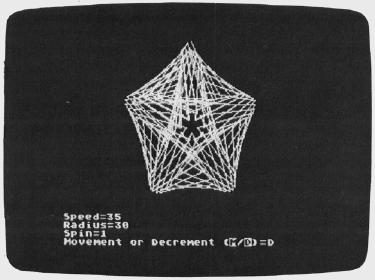"Spiralizer," Atari version.

This is actually the number of loops the pattern is going to have. You can choose an answer between -50 and 50. If you type 4 and RETURN, your pattern will have four complete loops. If you type -6 there will be six loops, but they'll be on the inner side of the pattern.

Use the back arrow to delete a character. If you simply press RETURN without typing in a number, the program will default to a value of 5, and will print it on the menu. Large numbers cause the program to draw straight segments, because of the large steps. The patterns thus produced are quite nice, too.

A pattern with three loops is easily understood, but what would a pattern with two loops look like? How about one loop? Could a pattern possibly have zero loops? Try them and see.

The second number you are asked to enter is the radius. This determines the ratio of the radii. You can choose any number between 1 and 60. A small number would make the inner radius small and the outer radius large, and vice versa. Like the first input, you can simply press RETURN and take the default value of 35.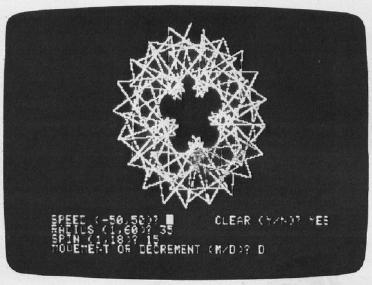Another Variation available in "Spiralizer," Apple version.

Next you are asked for the spin. An answer larger than 1 will make the pattern rotate while it is drawn, and, of course, the number of loops will change. You can choose between 1 (no spin) and 18. When spinning, the lines remain smooth and curvy, but it takes more time to draw the complete pattern. If you decide to quit while a pattern is being drawn, press any key and the program will return to the menu. To escape from the program, use RESET.

Now things become more complicated. You are asked, "MOVEMENT OR DECREMENT (M/D)?" If you choose M, the whole pattern will move while it is being drawn. If the spin is 1, the pattern will be drawn five times while it moves. If the spin is greater than 1, the pattern will move until it finishes rotating. If the spin is greater than 1 but less than 9, you will not be asked for this input.

Pressing D will cause the pattern to decrease in size while being drawn. The rules here are the same as above. If you press RETURN, the default value is NONE, and none of the above actions will take place.

 VIC, Atari, And Color Computer Notes Patrick Parrish, Editorial Programmer To create different spiral patterns with "Spiralizer," you must first type in several variables (speed, radius, etc.). Try different values for these variables or simply press RETURN (ENTER on the Color Computer) to use default values. For the VIC version of Spiralizer, you need the Super Expander cartridge. With this version, you can overlay as many as five spiral figures on the high-resolution graphics screen. The Atari and Color Computer versions allow you to overlay as many patterns as you wish. As with the Apple version, the Atari and VIC versions let you return to the beginning of the program by pressing any key if you are not pleased with the patterns being drawn.
 64 Notes Gregg Peele, Editorial Programmer Like the Apple, Atari, and Color Computer, the Commodore 64 is capable of high-resolution graphics. Unlike those computers, however, there are no specific commands in the 64's standard BASIC to create graphics on the high-resolution screen. At the time of this writing, the Video Support Package cartridge promised by Commodore is not yet available. We are left with a system of complex POKEs and formulas to accomplish simple hi-res graphics on the 64. Program 5, the Commodore 64 version, consists of routines to plot points, draw lines, and clear the hi-res screen. All these routines are in machine language and are accessed with the SYS command. The hi-res screen in these programs is located at 8192 (\$2000) and may be accessed from BASIC by two POKEs: ```POKE 53272, PEEK(53272)OR 8 POKE 53265, PEEK(53265)OR 32 ``` These POKEs put the screen in bitmap mode with a 320 × 200 high-resolution screen. To access the plot routine, LOAD and RUN Program 5, and set up a BASIC program which begins with the two POKEs previously mentioned. Next, POKE the X coordinate into 53242. If the X coordinate is greater than 255, then POKE 53243 with 1 and POKE 53242 with X - 256. If the X coordinate is less than 256, then POKE 53243 with 0. Now POKE the Y coordinate of the point into location 53240 and POKE a 0 into location 53241. Finally, SYS to location 49710 and your point will appear on the screen. To clear the screen, merely SYS 50039 and all points plotted will vanish. If you want to return to text screen, POKE 53272, 21 and POKE 53265, 27. If you want to draw a line between two sets of coordinates, then POKE the first end-point coordinate (X1) into 53220. As before, if the coordinate is greater than 255, then POKE 53221 with 1 and POKE 53220 with X1-256. If XI is less than 256, then POKE 53220, X1 and POKE 53221, 0. POKE the first Y coordinate into 53222 and POKE 53223 with 0. The coordinates of the other endpoint are now POKEd in starting with X2. If X2 is less than 256, then POKE 53224, X2 and POKE 53225,0. If X2 is greater than 255, then POKE 53224, X2 -256 and POKE 53225, 1. POKE Y2 into 53226 and POKE 53227 with 0. Once you have these coordinates in memory, use SYS49152 to plot your line on the high-resolution screen. To use "Spiralizer" on the 64, run Program 5 and then Program 6. Program 6 does all these POKEs for you and will create end�less variations of designs for you on the high-resolution screen.

Last, you are asked if you want to clear the screen. If you decide not to, then the new pattern will be drawn on the previous one. This feature allows you to make overlays of patterns.

For a nice sample, I suggest you try the following INPUTs: for speed, enter 7; for radius, 50; for spin, 18; then choose M for movement and clear the screen.

Experiment with different values, and you'll see some stunning designs.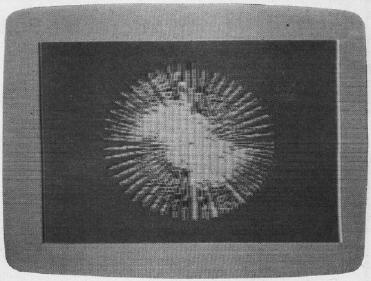A sunburst of color can be seen in the VIC version of "Spiralizer."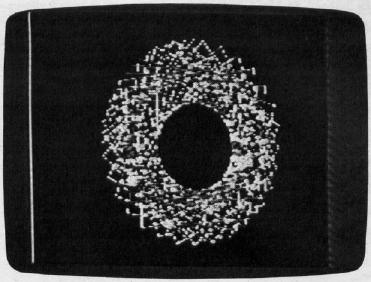"Spiralizer," Color Computer version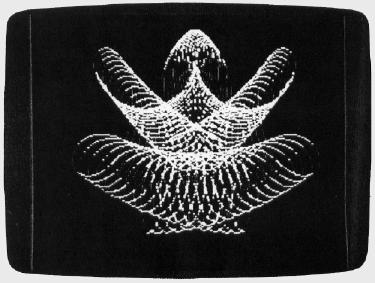Complex designs are possible with "Spiralizer," 64 version.

## Spiralizer — Apple version

```10  ONERR GOTO 90
60  R\$ = CHR\$ (8); HCOLOR= 3:H\$ = "" + R\$ + R\$ + R\$ + R\$
70  HOME : HGR
80  VTAB 9: HTAB 15: FLASH : PRINT "SPIRALIZER": NORMAL
90  POKE - 16368,0:Z = 5: HTAB 1: VTAB 21: PRINT "SPEED (-50,50)? ";:
GOSUB 380:K = Z
100 IF Z < -50 OR Z > 50 THEN 90
110 K = K - 1
120 Z = 35: PRINT "RADIUS (1,60)? ";:GOSUB 380: R = Z
130 IF Z <1 OR Z > 60 THEN VTAB 22: GOTO 120
140 R = R + 13:S = 1
150 Z = 1: PRINT "SPIN (1,18)? ";: GOSUB 380
160 IF Z < 1 OR Z > 18 THEN VTAB 23: GOTO 150
170 A = 1 / Z: IF Z > 1 AND Z < 9 THEN 240
180 SM = 1:M = 2: PRINT "MOVEMENT OR DECREMENT (M/D)? "H\$;
190 GET X\$: IF X\$ = CHR\$ (13) THEN M = 0:SM = 0: PRINT "NONE";: GOTO 240
200 IF X\$ = "M" THEN SM = 0: GOTO 230
210 I8F X\$ = "D" THEN M = 0: GOTO 230
220 GOTO 190
230 PRINT X\$;
240 VTAB 21:HTAB 24: PRINT "CLEAR (Y/N)? "H\$; GET T\$: IF T\$ < > "N" THEN PRINT
"YES": TEXT : CALL 62450: HGR
250 IF T\$ = "N" THEN PRINT "NO"
260 W = 1:Z = 139: IF M = 2 THEN Z = 80: IF A = 1 THEN W = 5:M = 1:Z = 122
270 IF SM - A = 0 THEN W = 5
280 IF A < 1 THEN K = K + A
290 C = 0.001: IF A < 1 / 9 THEN M = M / 2:c = C / 2
300 J = R:I = 79 - R
310 HPLOT Z,0
320 REM -MAIN LOOP-
330 FOR T = 0 TO 6.2831 / A * W STEP 0.06283:F = PEEK ( - 16384): POKE - 16368,0:
IF F > 127 THEN 90
340 IF SM THEN J = R * S:I = 79 * S - J:S = S - C
350 HPLOT TO Z + T * M - SIN (T) * J + SIN (T * K) * I,79 - COS (T) * J - COS
(T * K) * I: NEXT
360 GOTO 90
370 REM -INPUT SUBROUTINE-
380 L0 = 0:LI = 1:B\$ = ""
390 PRINT H\$;: GET A\$
400 IF A\$ = "-" AND L0 = 0 THEN PRINT A\$;:B\$ = A\$:L0 = 1:L1 = 2: GOTO 390
410 IF A\$ = CHR\$ (13) AND L0 > 0 THEN Z = VAL (B\$): PRINT : RETURN
420 IF A\$ = CHR\$ (13) THEN PRINT Z: RETURN
430 IF A\$ = R\$ AND L0 > 1 THEN PRINT A\$;:B\$ = LEFT\$ (B\$, LEN (B\$) - 1):
L0 = L0 - 1: GOTO 390
440 IF A\$ = R\$ AND L0 = 1 THEN PRINT A\$;:B\$ = "":L0 = 0: GOTO 390
450 IF L0 > L1 THEN 390
460 IF A\$ < "0" OR A\$ > "9" THEN 390
470 PRINT A\$;:B\$ = B\$ + A\$:L0 = L0 +1: GOTO 390
```